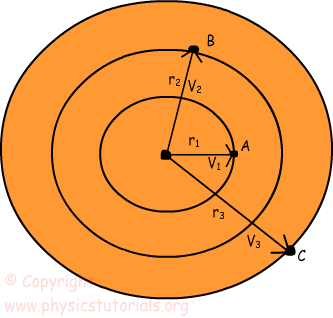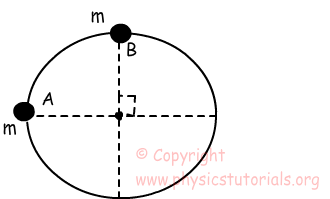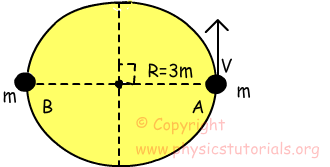# Tangential Speed Velocity with Examples

Linear Speed (Tangential Speed):

Linear speed and tangential speed gives the same meaning for circular motion. In one dimension motion we define speed as the distance taken in a unit of time. In this case we use again same definition. However, in this case the direction of motion is always tangent to the path of the object. Thus, it can also be called as tangential speed, distance taken in a given time. Look at the given picture and try to sequence the velocities of the points larger to smaller.In a given period of time all points on this rotating object have same revolutions. In other words, if A completes one revolution, then B and C also have one revolution in a same time. The formula of the speed in linear motion is;

Speed=distance/time

As I said before, speed in circular motion is also defined as the distance taken in a given time. Thus, speeds of the points given in the picture below are;

V =Distance/time If the object has one complete revolution then distance traveled becomes; 2πr which is the circumference of the circle object.

VA=2πr/time

Period: Time passing for one revolution is called period. The unit of period is second. T is the representation of period.

The equation of tangential speed becomes;

VA=2πr/T

Frequency: Number of revolutions per one second. The unit of frequency is 1/second. We show frequency with letter f.

The relation of f and T is;

f=1/T

Now; with the help of the information given above lets’ sequence the velocities of the points on given picture.

Since the velocity or speed of the points on rotating object is linearly proportional to the radius r3>r2>r1;

V3>V2>V1 To sum up, we can say that tangential speed of the object is linearly proportional to the distance from the center. Increase in the distance results in the increase in the amount of speed. As we move to the center speed decreases, and at the center speed becomes zero. We use the same unit for tangential speed as linear motion which is “m/s”.

Example: A particle having mass m travels from point A to B in a circular path having radius R in 4 seconds. Find the period of this particle.Particle travels one fourth of the circle in 4 seconds. Period is the time necessary for one revolution. So,

T/4=4s

T=16s.

Example: If the particle having mass m travels from point A to B in 4 seconds find the tangential velocity of that particle given in picture below. (π=3)We first find the period of the motion. If the particle travels half of the circle in 4 seconds;

T/2=4s

T=8s

v=2 π R/T

v=2.3.3m/8s=9/4 m/s tangential speed of the particle

Rotational Motion Exams and Solutions